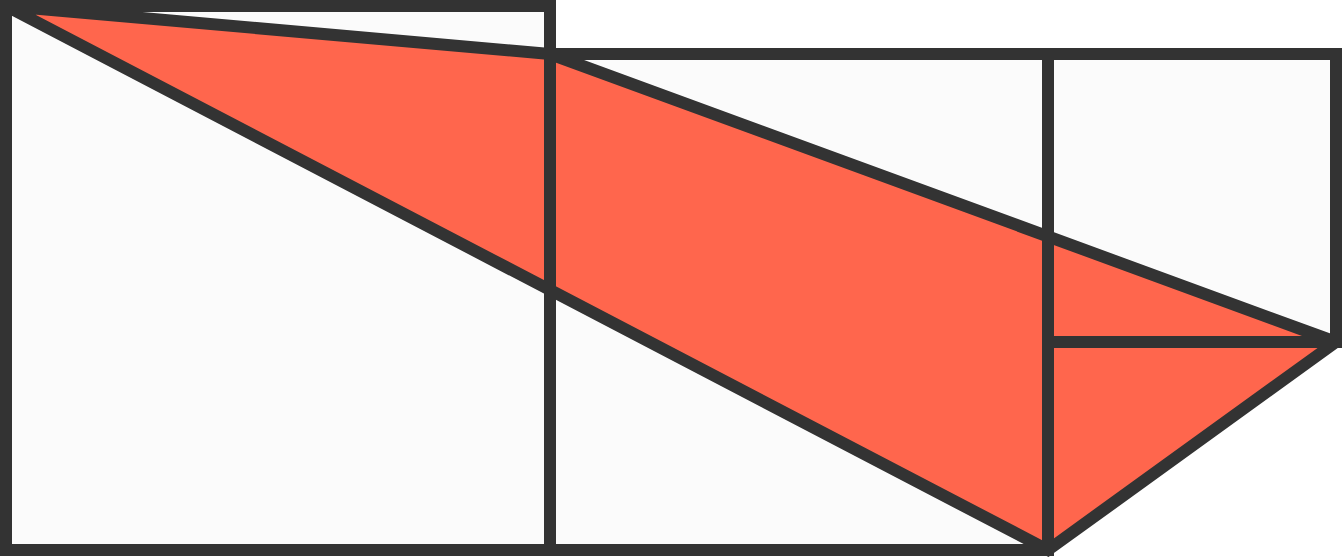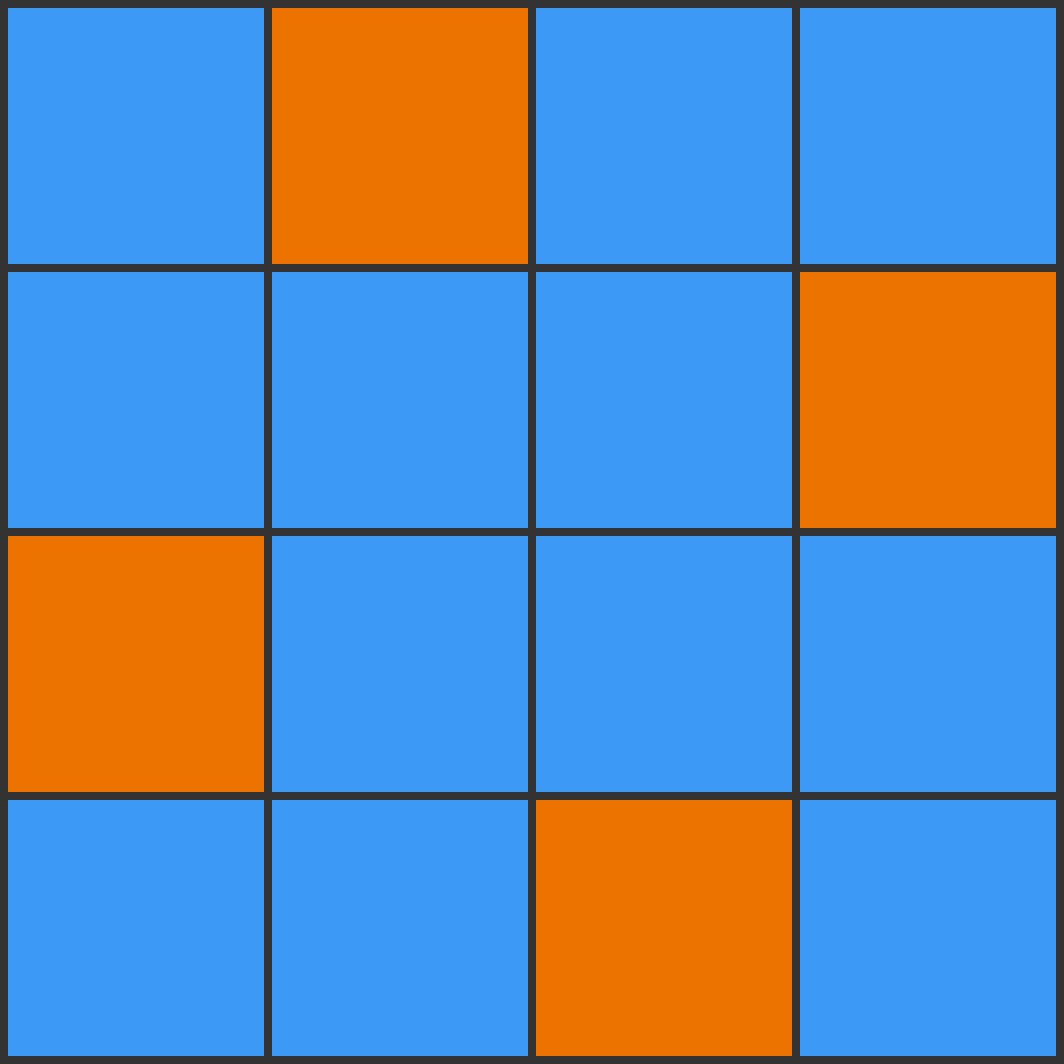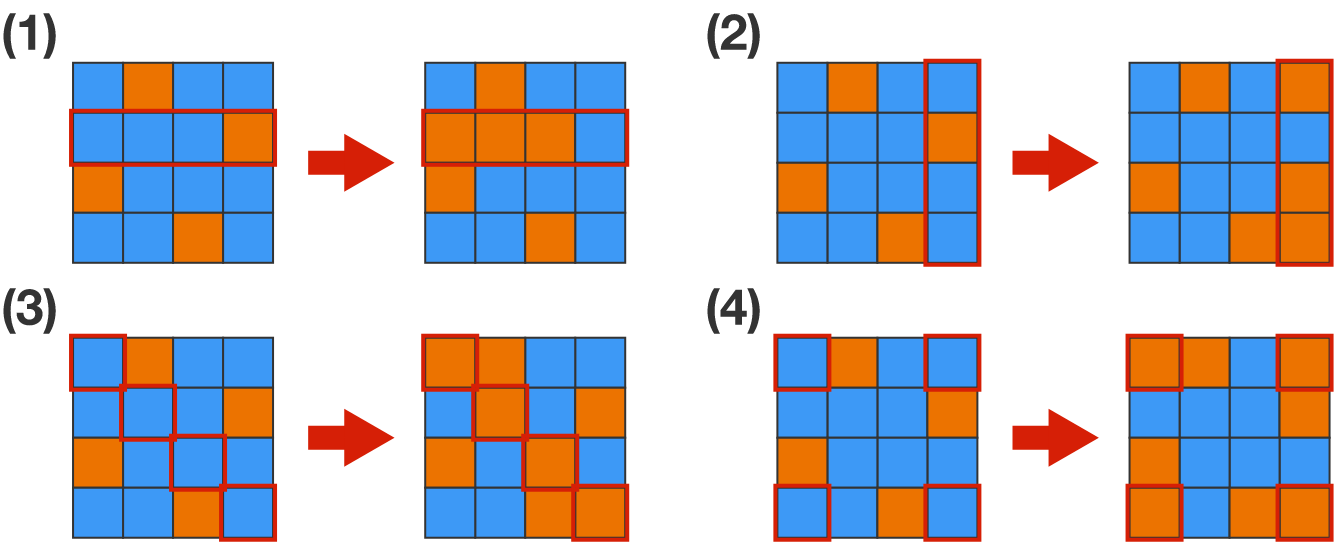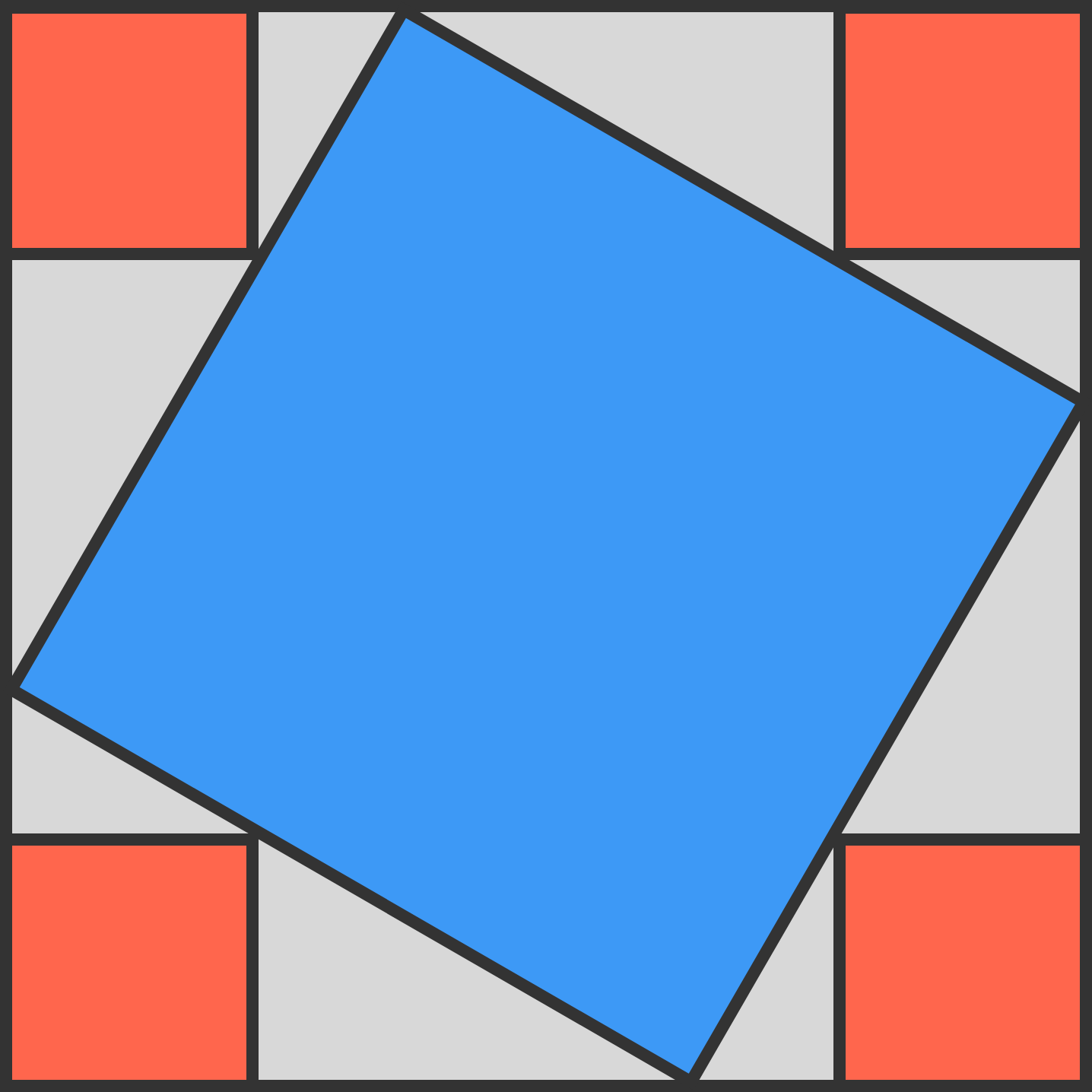# Problems of the Week

Contribute a problem

# 2018-07-23 Intermediate

Three squares of different areas are side by side. The middle square’s area is 16, and its opposite sides are each collinear with an adjacent square’s side.

What is the area of the red quadrilateral?$a$ and $b$ are positive integers such that $a^2+b^2$ is divisible by $7.$

Are both $a$ and $b$ always divisible by $7?$On the $4\times 4$ board above, each tile is colored orange on one side and blue on the other. With each move, you must flip one of the following: (1) an entire row, (2) an entire column, (3) an entire diagonal, or (4) all four corners.

After some number of moves, can you make the whole board orange?With each move, you must flip a row, column, diagonal, or flip the four corners.The blue square touches each side of the largest square, filling $\frac{29}{50}$ of it.

Each of the 4 red squares at the corners of the largest square touch the blue square.

The fraction of the largest square that is red is $\frac{a}{b},$ where $a$ and $b$ are positive coprime integers.

What is $a+b?$

$\large {9^{{}^9}}^{{}^9} \qquad {{9^{{}^9}}^{{}^9}}^{{}^9}$

Are the last two digits of these numbers the same?

×